Electronic Circuits Trainer　ITF-01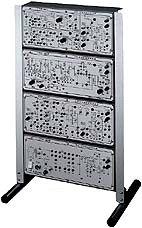The lTF-01 is a trainer for practically mastering various circuits becoming the basis of electronic circuits through training and is composed of the following four units. Since a radio receiver is made composed by combining the ITF-012, 013 and 014 among those units, overall understanding can be made by the whole training as well as understanding of basic circuits.When 4 units abovementioned are assembled in the rack mount ITF-015, then overall training  (exercises) as a radio receiver can be performedThe increase of understanding the amplifier circuits becoming the most basis of electronic circuits  is taken into consideration, so there3 is an effect as the fundamental knowledge training unitAn example of the standard basic operation of each circuit is made possible only by integrated circuits,  but on the other hand, constants designed by trainers are also possible to be attached, so interest  in the unit is excited, furthermore education effect can be measuredThe training unit ITF-014 is also made operated as the power source of the whole these units,  so the external power source is unnecessary

Contents of Training

 1. Oscillator and Modulator Circuits Training Kit   lTF-011 +Training in Collector tuning (bias, frequency and oscillation requirements) +Training in CR-type phase transient oscillators +Training in collector modulating circuits 2. AM Receiver Circuit Training Kit  lTF-012 +Training in IF tuning characteristics +Training in bias characteristic of AGC and non-AGC +Training in detection characteristics +Training in Receive Circuits and Frequency Converters 3. Audio Amplifier Circuit Training Kit  ITF-013 +Training in bias determination, amplitude, frequency characteristics, phase characteristic wide band  amplification (training in feedback, peaking and low frequency boost) +Training in double amplifier circuits +Training in power amplifier circuits (bias, power, efficiency, distortion) 4. Rectifier and Smoothing Circuit Training Kit  ITF-014 +Training in half-wave rectifier circuits and full wave rectifier circuit +Training in smoothing circuits(C. L.C. pi) 5．Radio receiver

Logic Circuits Trainer　ITF-02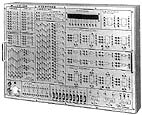The lTF-02 is a trainer, by which leaning can be made visually by combining the undermentioned units, which is provided with each circuit element, signal source and indicator on its panel so that training can easily be performed from the foundation to the application of logical circuits.Since each circuit element is indicated graphically on its panel so as to understand them easily,  the elements can easily be understoodThe level of input/output can be indicated as  and  by LED digit indicator on the panelSince input/output terminal is attached to each circuit element, the connection between circuit  element is possible and various combinations can be madeSince the number of circuit element is many (45 gates, 10 flip-flops), application  is made sufficientlySince a signal source is contained, most of training can be performed without the  external generator

Contents of TrainingBasic operation training of AND, OR, NOT, NAND, NOR, and Exclusive ORAssembly and operation training of half-adder and full-adderAssembly and operation training of encoder and decoderBasic operation training of R-S and J-K flip-flopsBasic operation training of shift registers, other application trainin

Pulse Circuits Trainer　ITF-03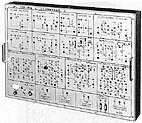The ITF-03 is trainer, by which leaning can be made visually by combining the under mentioned units, which is provided with each circuit element and signal source on its panel so that training can easily be performed from the foundation to the application of pulse circuitsSince each circuit element is indicated graphically on its panel, the elements can be understoodSince constants designed by trainees are possible to be attached also, comparison with  standard operation, the change of operation caused by constant change, etc., can be observed,  then it is easy to rasp the applicability and understanding of the traineesSince each circuit is provided with input/output terminals, the connection between circuits is  possible, and various combinations can be madeSince the number of circuit element is many (13kinds), application is made sufficientlySince a signal source is contained, even through the external generator is no used specially,  most of training can be performed

Contents of TrainingTraining of differentiation circuit and integration circuitTraining of clipping circuit and clamping circuitTraining of logic circuits (AND, OR, NOT)Training of Miller circuit and bootstrap circuitTraining of multi-vibrator (mono-stable, astable, bistable, Schmitt trigger)

Semiconductors Trainer　ITF-05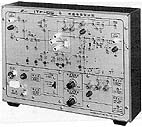The ITF-05 is a trainer which is designed to master the operation and characteristic of various semiconductor elements, by which two-terminal and three-terminal semiconductors can be mastered.This is a training unit for general use, by which various two-terminal and three-terminal  semiconductor elements are possible to be measuredSince various source (current and voltage) controlling the control electrodes (base of transistor,  training is gate of FET, gate of SCR, etc.) of three terminal element is integrated, when the same kind of  performed at the same time, not so many external sources are requiredTwo-terminal type power semiconductor can be mastered by using the self contained voltage sourceIn the training of the transistors, the AC measurement of h parameter is also possibleThis is designed so that it may be protected from circuit shirting, miss-swiring, etc.

Contents of TrainingTraining of characteristic (voltage and currant) measurement of two-terminal elements Measurements : diode, zener diode, selenium rectifier, varistor, thyristor, etc.Training of characteristic (voltage, current, various parameters) measurement of three-terminal elements Transistor : static characteristic, h parameter (AC overlapping) FET : static characteristic, amplitude, impedance SCR : static characteristic LED : static characteristic

Electronic Counter Circuits Trainer　ITF-06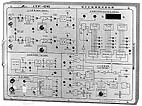The ITF-06 is a trainer, by which leaning can be made visually by arranging each circuit on its panel and combining them in order to understand the basic operation of the electronic counterSince each circuit block is indicated on its panel graphically, the circuits are easy to be understoodSince each circuit block is provided with input/output terminal. circuit configuration for  the each function can be made easilyTraining can be performed for 5 functions (frequency measurement, period measurement,  time interval measurement, frequency ratio measurement, totalizaing measurement)Since the measured results are not only indicated but also possible to be output as digital data,   this unit can be used as A/D converter

Contents of TrainingTraining of frequency measurementTraining of period measurementTraining of time interval measurementTraining of frequency ratio measurementTraining of totalizing measurement

Arithmetic Calculator Circuits Trainer　ITF-07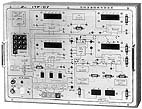The lTF-07 is a trainer which is designed so that training of four arithmetic operations can be performed while trainees confirm visual operations one by one by indicating the basic circuits of calculator on the panelSince the circuit block having necessary function for four arithmetic calculation  are graphically and respectively indicated on the panel respective, the circuit is easy composed and understoodEstablishment of input is performed by decimal and calculated by binary, but its result  can be indicated by decimal also as well as binaryThe content of registers is indicated as  and  by LED digit indicatorSince indication is made by counting shift clock pulse, mistakes caused by  misunderstanding of trainees can be avoided

Contents of TrainingTraining of adding calculatorTraining of subtracting calculatorTraining of multiplication calculatorTraining of dividing calculator